# Physics 1 A Section 6 October 16 2008

• Slides: 19Physics 1 A, Section 6 October 16, 2008Section Business • Quiz #1 was due 3 hours ago! • Homework and quiz solutions will be posted on-line on the course web page.T. M. U. Problem 6. 4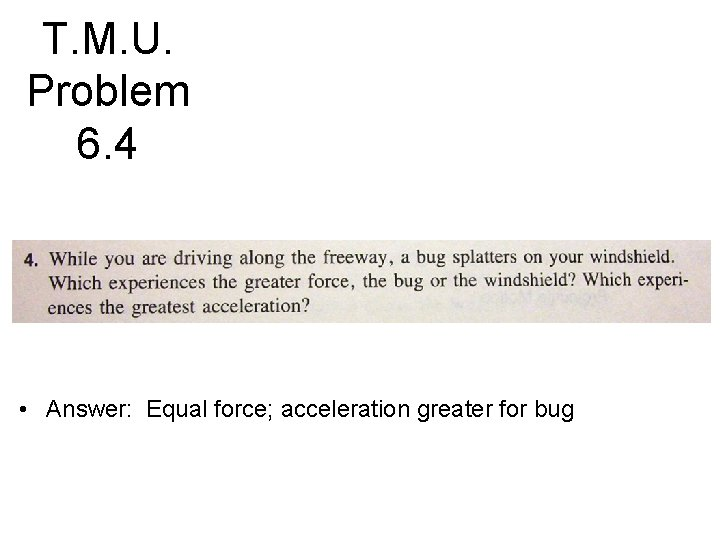T. M. U. Problem 6. 4 • Answer: Equal force; acceleration greater for bugT. M. U. Problem 6. 1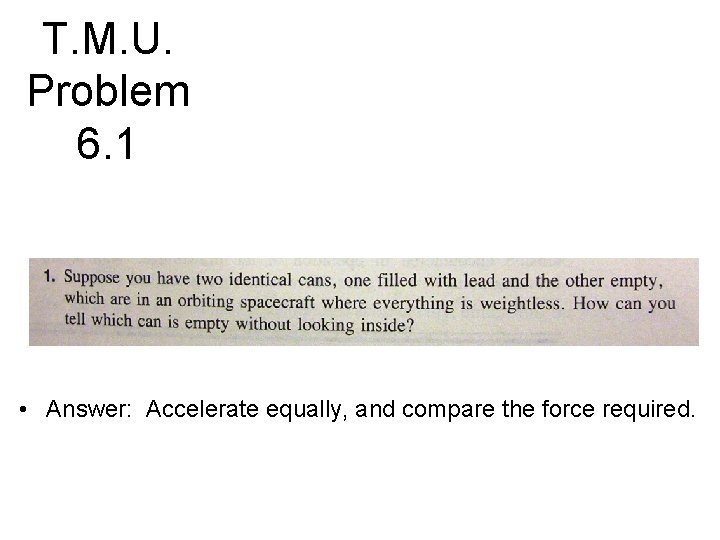T. M. U. Problem 6. 1 • Answer: Accelerate equally, and compare the force required.T. M. U. Problem 6. 5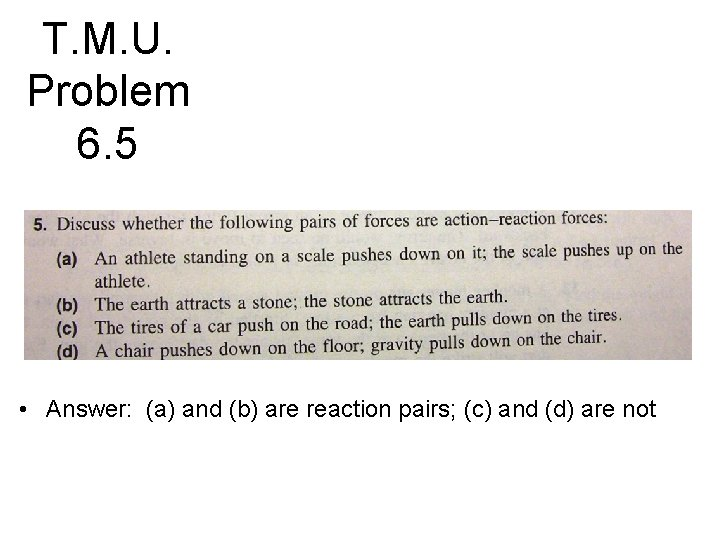T. M. U. Problem 6. 5 • Answer: (a) and (b) are reaction pairs; (c) and (d) are not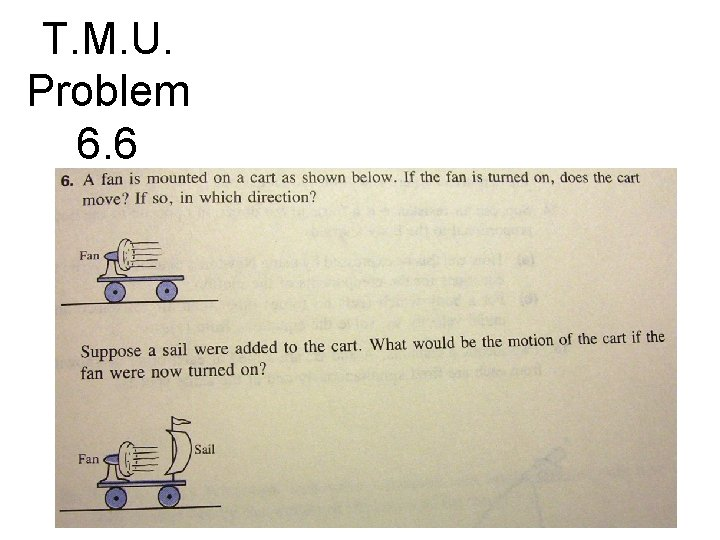T. M. U. Problem 6. 6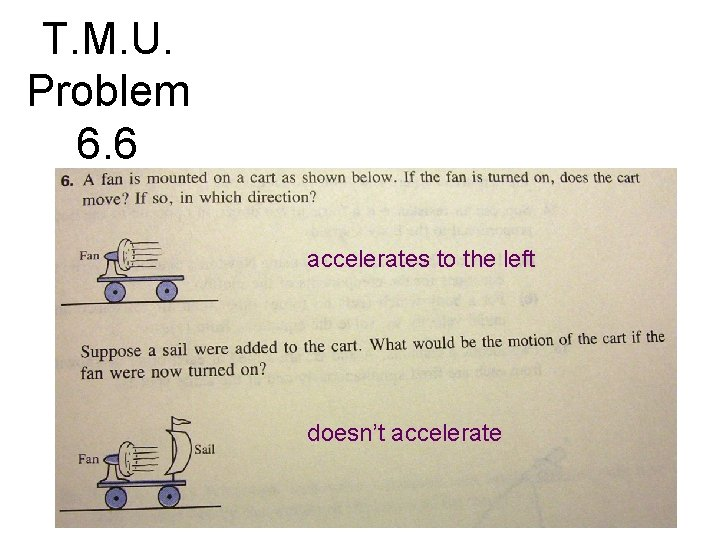T. M. U. Problem 6. 6 accelerates to the left doesn’t accelerate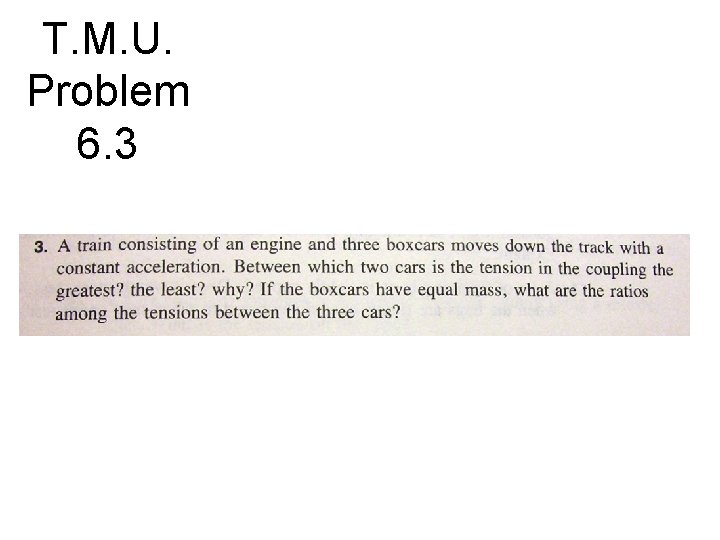T. M. U. Problem 6. 3T. M. U. Problem 6. 3 • Answer: Tension greatest between engine and front boxcar. Tension is 3 ma, 2 ma, ma from front to back.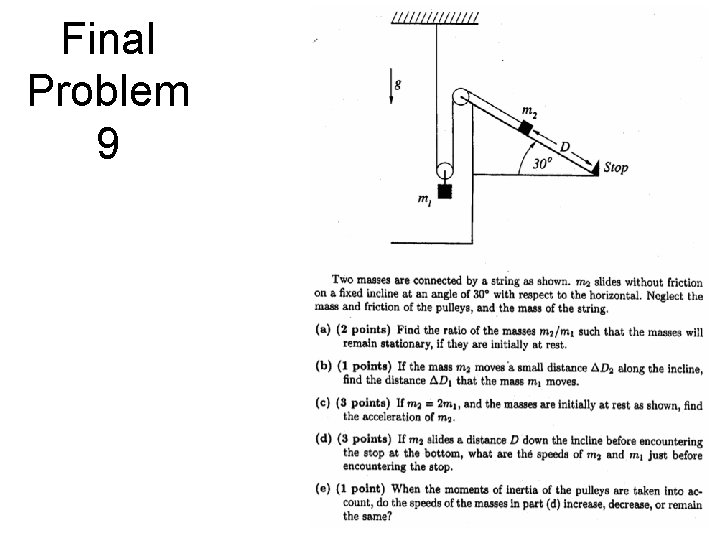Final Problem 9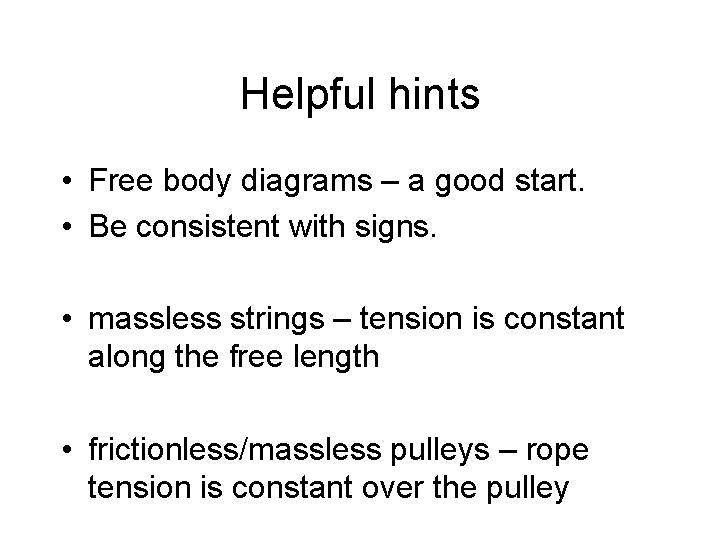Helpful hints • Free body diagrams – a good start. • Be consistent with signs. • massless strings – tension is constant along the free length • frictionless/massless pulleys – rope tension is constant over the pulley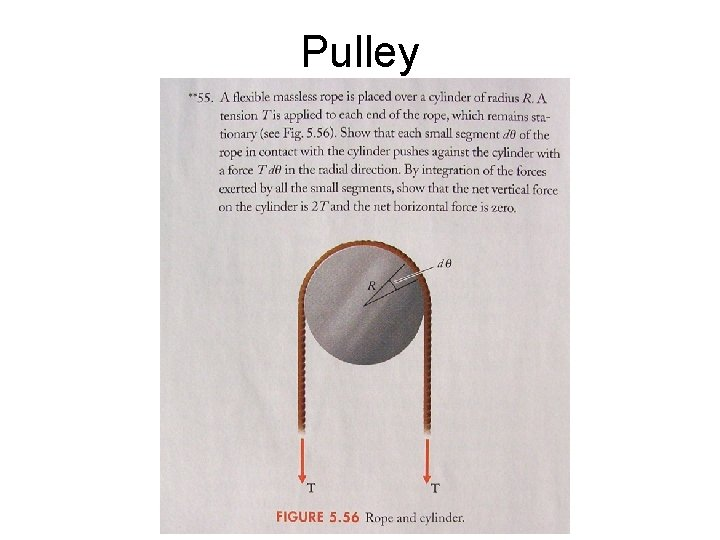Pulley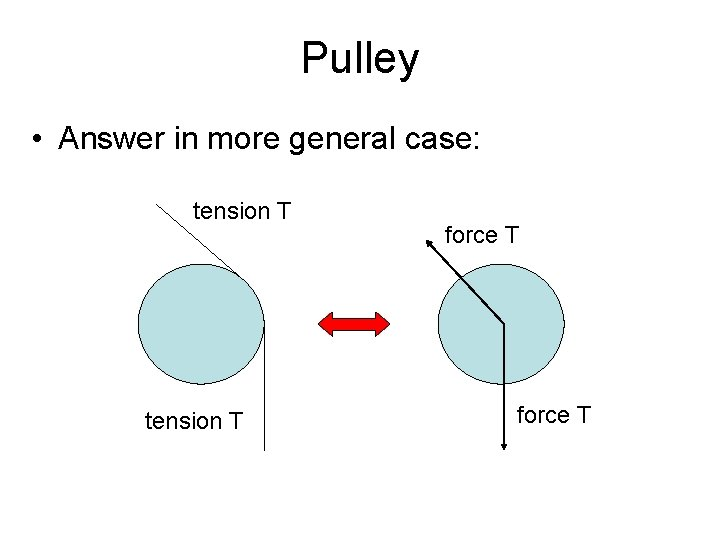Pulley • Answer in more general case: tension T force TFinal Problem 9 • Answer: a) m 2/m 1 = 1 b) DD 1 = DD 2/2 c) a 2 = 2 g/9 d) v 2 = 2/3 sqrt(g. D), v 1 = 1/3 sqrt(g. D) e) decreaseMonday, October 20: • a gravity/circular orbit problem of some sort • Quiz Problem 37 • Optional, but helpful, to look at these in advance.T. M. U. Problem 6. 2